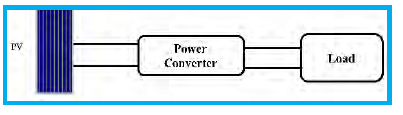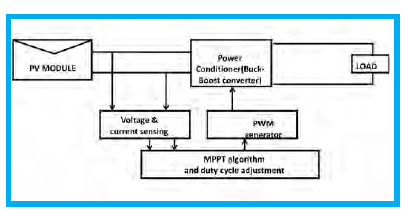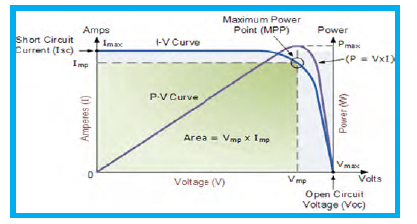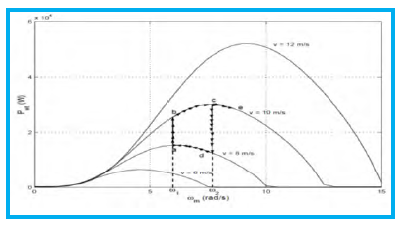# MPPT Techniques for Renewable Energy Systems

The various aspects of various maximum power point tracking (MPPT) techniques utilised for different renewable energy systems in terms of their needs and advantages are reviewed in this article.

The power characteristics of renewable energy sources is nonlinear in nature, there exists one operating point where they generate maximum power. In order to take full advantage of available energy resource and achieve maximum utilisation efficiency, maximum power point tracking control techniques which extracts maximum power from the renewable source is essential. Article highlights various MPPT techniques for solar photovoltaic (SPV) and wind energy conversion system.

Promotion of renewable energy technologies is fundamental for economic growth and sustainable development of the country. Renewable energy sources are clean, green, abduntaly available and can easily be replenished. Renewable energy sources when utilised for power generation helps to improve system efficiency, reliability and helps to relive stress on power transmission and distribution infrastructure helping in reducing power deficit. The issues with renewable energy systems used for power generation are intermittent and variable nature of these sources, large area requirement to harness power, despatchibility, low conversion efficiency and capacity factor. For growth of this sector maximum utilisation of the available renewable source with high conversion efficiency should be targeted. Since renewable sources have non linear power characteristics, there exists one optimum point where power generation is maximum. Locating and tracking this maximum power point is essential for utilising the source to optimum available level and achieve maximum utilisation efficiency. This article discusses the need, methods, advantages of various maximum power point tracking (MPPT) techniques utilised for different renewable energy systems.

Maximum power point tracking for Solar Photovoltaic Systems Need for power tracking in SPV arises because of the rotation and revolution of earth, changing environmental conditions, nonlinear I-V characteristics. Tracking methods are both mechanical and electrical. In mechanical tracking, due to rotation and revolution of earth SPV panels are made to track movement of earth with respect to sun to ensure maximum insolation is received by PV systems. Trackers can be either two-axis, which track sun in both in azimuth and altitude angle so the PV module always points directly to sun or single axis which track only one angle.

Electrical tracking: Power-voltage and Current-voltage characteristics depend upon insolation, temperature, shading, environmental conditions, load connected. Electrical MPP tracking means to make operating point coincide with point where maximum power occurs. Make load characteristics to adjust such that it intersects I-V and P-V characteristics at MPP. Current from SPV module depends on insolation and temperature, I-V characteristics of module will change whenever there is change in Insolation and temperature. This in turn result in change in maximum power point which may not coincide with operating point of load and hence maximum power will not be transferred to load. PV cell have single operating point where value of (I) and (V) of module result in maximum power output. This value corresponds to a particular resistance which is equal to V/I as per ohms law. SPV module has exponential relationship between I and V. Maximum power point occurs at knee of curve where resistance is equal to negative difference of resistance (V/I= -dV/dI). A MPPT is power electronic DC-DC converter embedded with algorithm which is inserted between PV module and load to achieve optimum matching. Purpose of MPPT system is to sample the output of PV cells and apply proper resistance (load) to obtain maximum power for any given environmental condition. To SPV module DC to DC converter acts as load and hence input resistance of converter is of interest. In Rin of converter lies on Vmp- Imp point, maximum power can be transferred to the converter and in turn to load.Figure 1:- SPV system with Power converter

For Buck converter Rin= Ro/D2 mp, where Ro is load resistance, Rin input resistance of converter
For Buck boost converter Rout (Module)= Rin = Ro(load). (1- Dmp)2/ D2mp
Ro (load) cannot be changed, RHS quantity can be changed to Rout (Module) by changing value of Duty cycle (Dmp) through feedback network. By changing duty cycle resistance seen by source changes.

MPPT system tracks maximum power point of PV cell/module and adjusts Dmp from the feedback network based on voltage and current of SPV module to match the load. Input resistance of power electronic converter has to be equal to solar cell output resistance at MPP. Special control technique has to be developed for converter to satisfy such condition.Figure 2:- SPV system connected to load with MPPT system

Algorithms for SPV MPPT system
They are used to control duty cycle of converter or power conditioning circuit connected between SPV and load to extract maximum power from source and fed it into load. Their main mission is to adopt the operating point of module usually modifying its output voltage in order to adapt the system to different irradiance and temperature variations.
Choice of MMPT technique is decided by following factors:
i) Implementation complexity
ii) Number of sensors required
iii) Ability to detect local maxima
iv) Cost
v) Response time
vi) Application.

Maximum power point can be estimated by both – Direct method, and Indirect method.

In indirect method, MPP is estimated from Voltage, Current, Insolation, and Using empirical data. Various techniques which can be used are: Curve fitting, Look up table, Fractional open circuit, and Fractional short circuit.

Direct method uses voltage and/or current information, prior knowledge of PV panel is not required various techniques which can be used are: Hill climbing/ Perturb and observe; Incremental conductance; Fuzzy logic; and Slide control.
Fractional Open circuit voltage method: Operating point of SPV array is kept near MPP by regulating array voltage and matching it to fixed reference voltage Vref.
Fractional short circuit method: Operating point of SPV array is kept near MPP by regulating array current and matching it to fixed reference current Iref.
Perturb and Observe algorithm: P&O algorithm continuously perturbs the operating point of system causing PV array terminal voltage to fluctuate around the MPP voltage even if solar irradiance and cell temperature varies. P&O algorithms operate periodically perturbing (i.e. incrementing or decrementing) the array terminal voltage and comparing PV array output power with that of previous perturbation cycle. If the PV array operating voltage changes and power increases (dP/dV>0), control system moves the PV array operating point in that direction, otherwise operating point is moved in the opposite direction.Figure 3: Solar PV and IV characteristics

Incremental Conductance Algorithm: This algorithm decrements or increments PV array voltage Vpv to track new MPP. It is based on the fact that the derivative of output power with respect to panel voltage is equal to zero at maximum power point. It is based on the fact that the slope of PV characteristics is zero at MPP, Negative on right of MPP and positive on left of MPP.

MPPT for Wind Energy Conversion Systems
At a given wind speed, the mechanical power developed by wind turbine is function of shaft speed. The wind turbine mechanical power output is affected by ratio of wind turbine shaft speed and the wind velocity that is Tip speed ratio (λ). As a result of variations in wind velocity, turbine shaft speed or generator shaft speed and wind turbine power PT will change. Shaft speed ωpmax corresponding to maximum power is obtained from equation dPT/dPmax=0. From mechanical design point of view, wind turbine are designed to operate at maximum power for an average wind speed. However wind speed does not stay at an average level all the time. At lower wind speeds, TSR (λ) is increased with constant rotor speed and accordingly power coefficient Cp decreases.

As a consequence wind turbine is not operating under optimal conditions (where Cp is low) most of the time.

There are three methods by which MPPT can be adopted for wind turbine system.

Tip speed ratio control: Both wind speed and turbine speed are measured or estimated to maintain TSR at optimum level λopt so that maximum power is extracted.Figure 4: Power coefficient v/s Tip speed ratio  characteristics at different wind speeds

Perturb and Observe Method: It is based on perturbing the turbine shaft speed in small steps and observing resulting changes in turbine mechanical power. If the shaft speed increment (ΔωT) results in a turbine power increment (ΔPT), operating region is in uphill curve and increase shaft speed must be continued toward MPP otherwise operating is downhill curve, so the shaft speed should be decreased by P&O method to reach MPP. Till the maximum power point is reached i.e (ΔPT/ ΔωT=0).Figure 5: Maximum power point characteristics for wind
turbine systems

Power Signal feedback method: Power is measured and controlled to reach maximum power according to power curve of wind turbine is obtained. In this method maximum power is tracked by shaft speed measurement only and no wind velocity measurement is required.

Conclusion
The various aspects of various maximum power point tracking (MPPT) techniques utilised for different renewable energy systems in terms of their needs and advantages  are  reviewed in this article.Jayesh G. Priolkar,
Asst Prof in E&E Dept,
Goa College of Engineering,
Farmagudi Goa